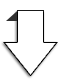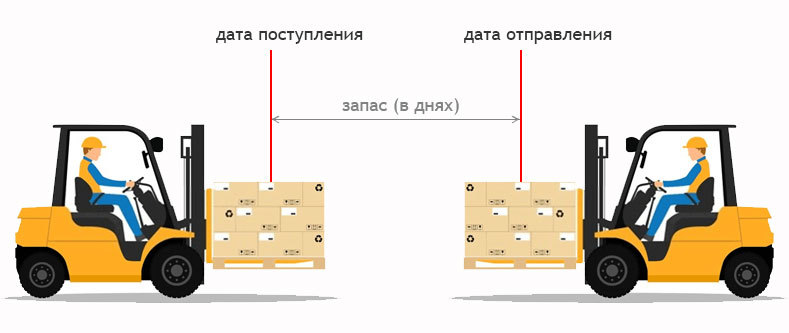Economy + ...THIS     БОЛЬШЕ ЧЕМ ЭКОНОМИКА
Персональная помощь в решении задач экономики и WEB-разработки
Rus Eng
BUTW - Become more Useful in This World !
Be in the topic   -    SUBSCRIBE      ECONOMY IN SIMPLE +   в TelegramYour information на KAKTOTAK.BY    Cooperation - on an ongoing basis    Individual offers
Simple online solutions to problems of economics and finance

# The working capital reserve rate in days – how to count ?What is working capital and from what types of current assets it is formed, we have already considered.

What is the principle of calculating the working capital reserve rate based on, i.e. the main criteria on which its need and economic efficiency depend.

To begin with, let's find out what is the reserve rate of a current asset in days.

The stock rate is the period when the current asset is in a "frozen state" from the moment of its formation to the moment of transition to another form.

For example, materials for the production of products are one of the types of current assets.

The period from the moment it arrives at the warehouse to the moment it is transferred to production (when it will switch to another form of WIP) it will be in a stationary state – «frozen». This number of days is determined by the norm of the stock of this material.Similarly, the stock rate for work in progress (WIP), finished products and accounts receivable is determined.

How to calculate the stock rate for the current production.

The main one for calculating this indicator in days is analytical data (a turnover sheet for a certain type of asset) and synthetic accounting (balance sheet) for certain dates, as well as some forms of statistical reporting.

Consider a concrete example.

It is necessary to determine the average norm of the stock of materials of the enterprise based on the results of its work for 2020 (365 days).

• we determine the average annual size of the remaining materials in the warehouse, based on the balance sheet data for the 1st day of each quarter of 2020 and divide by 4
(37 500 + 41400 + 39 650 +38 700)/4 = 39& nbsp;313 rubles.
• we determine from the statistical form 4-f how many materials were written off in the cost (production) of products for 2020.
430,000 rubles
• we determine how many times on average per year (365 days) the material turned around:
430 000 / 39 313 = 11 times
• we calculate the material stock rate based on the calculated turnover:
Since the estimated period is a year, we take 365 days for the calculation.
365 /11 = 33 days
That is, on average, the material is in stock in a stationary state for 33 days.

The calculated stock rate indicator determines the period of "deadening" of the asset and its liquidity, and can serve as a basis for planning working capital for the enterprise for a long-term period, based on projected revenue volumes, cost and other indicators.

The turnover indicator (number of revolutions) is the inverse indicator of the stock rate (in days). Improving the efficiency of working capital is reduced to the need to increase the number of revolutions of the asset over the period and a corresponding decrease in its stock rate in days.

Online calculation of the stock rate and turnover for any type of current asset can be done using our calculator.

Working capital stock rate in days – how to count ? - Something Like That!

Если у вас есть какая-либо потребность в решении определённой задачи, связанной с экономикой, финансами или web-разработкой     telegram или форма обратной связи

136
0
Name
E-mail
Rating
Review

Конвертер валют
RUBUSDEURCNYBYNSHORT - о чём речь
ECONOMIC LITERACY